# RF Tutorial Lesson 13: Investigating RF Transmission of Digital Data

 Tutorial Project: Investigating RF Transmission of Digital DataObjective: In this project, you will transmit various pulse waveforms over a long transmission line and investigate the signal distortion effects. Concepts/Features: Transmission Line Attenuation Constant Pulse Waveform Exponential Waveform Digital Source DAC Conversion Bridge Pulse Width Modulation Ramp Generator Nonlinear Controlled Source Minimum Version Required: All versions

## What You Will Learn

In this tutorial you will explore the transient response of long transmission lines when excited with digital signals. You will define a digital source to perform a mixed-mode digital-RF simulation and will transmit the binary output of a pulse width modulator (PWM) over the long transmission line. You will also learn how to define complex waveforms in RF.Spice A/D.

## RF Signal Transmission Over Long Distances

The following is a list of parts needed for this part of the tutorial lesson:

Part Name Part Type Part Value
VS Voltage Source Waveform: TBD
XTL1 Generic T-Line Defaults: Z0 = 50, eeff = 1, len = 1500
XTL2 Generic T-Line Defaults: Z0 = 70.71, eeff = 1, len = 37.5
RS Resistor 50
RL Resistor 100

In RF Tutorial Lesson 2, you analyzed the transient response of a quarter wave transformer that was designed to match a 100Ω load to a 50Ω transmission line connected to the voltage source with a 50Ω source resistance at an operational frequency of 2GHz. For this tutorial you are going to use the same circuit but with a much longer transmission line.

Place and connect the parts as shown in the figure below:The Quarter-Wave Impedance Transformer circuit connected to a long transmission line segment.

Define a pulse waveform for the voltage source VS according to the table below:

 Initial Voltage 0 1 0 10p 10p 250p 500p

Run a Transient Test of this circuit with the parameters specified below:

 Start Time 0 20n 1p 1p v(2), v(4)

The results are shown in the figure below. Due to the very long length of the 50Ω T-Line XTL1, the source signal initially sees an impedance match at Node 2. The peak amplitude of v(2) is therefore 0.5V. The time it takes the incident propagating TEM wave to reach the load is:

$t_L = \frac{L_{tot}}{c} = \frac{1.5 \text{m} + 0.0375 \text{m}} {3\times 10^8 \text{m/s} } = 5.125\text{ns}$

You can see from the figure that v(4) starts at about t = 5.1ns. As we discussed in RF Tutorial Lesson 2, the impedance matching property of the quarter-wave transformer is valid only at 2GHz. The other harmonics of the pulse signal don't see a perfect match condition. As a result, there is a reflected signal, which takes another 5.125ns to reach Node 2. You can see that after t = 10.25ns, the voltage at Node 2 starts to get distorted due to superposition of the reflected signal.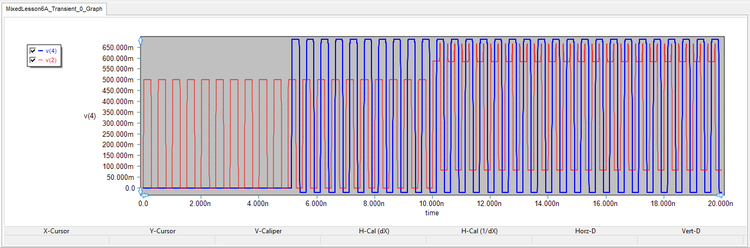The graphs of input voltage v(2) and output voltage v(4) as a function of time.

## Exploring a Lossy Transmission line

So far you have worked mostly with lossless transmission lines with a zero attenuation constant. In this part, you will use a long lossy transmission line segment. Open the property dialog of the T-Line XTL1 and set the value of the Alpha parameter to 1dB/m. For your 1.5m line, this means a total attenuation of 1.5dB.Adding losses to the transmission line by defining a nonzero attenuation constant.

Run another transient test with the same parameters s before. The results are shown in the figure below. The line attenuation causes additional distortion of the transmitted signal. Also, in comparison to a steady value of 687mV in the previous lossless case, you can see that the peak amplitude of the load voltage has now dropped to a value of 578mV at t = 5.2ns and continues to decline to a value of 528mV at t = 20ns. You can easily verify your results noting that

$\frac{v_L^{lossy}}{v_L^{lossless}} = 20\log \left( \frac{578mV}{687mV} \right) = 20\log(0.841) = -1.5dB$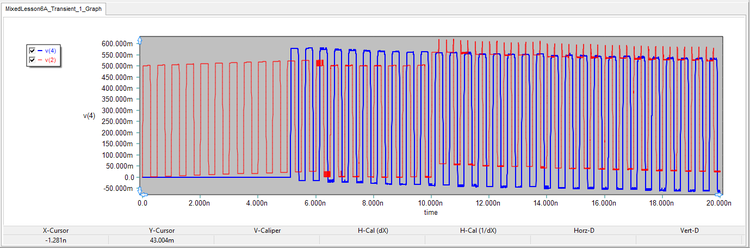The graphs of input voltage v(2) and output voltage v(4) after adding losses to the long transmission line segment.

## Using a Digital Source to Build a Mixed-Mode Circuit

In this part of the tutorial lesson, you will use a digital source to replace the analog voltage source of your transmission line circuit. A digital source generates a sequence of binary bits, 0's and 1's, as a function of time. You can access a digital source using the menu item Menu > Parts > Digital I/O > Digital Source or using the keyboard shortcut Alt+D. You have to define the bit sequence in the "Time-Value Array" table in the property dialog of the digital source. Enter the following data for the excitation of your circuit over the time interval [0 - 10ns]:

Time State
0 0
250ps 0
260ps 1
500ps 1
510ps 0
900ps 0
910ps 1
1250ps 1
1260ps 0
1500ps 0
1510ps 1
2000ps 1
2010ps 0
2500ps 0
2510ps 1
2750ps 1
2760ps 0
3000ps 0
3010ps 1
3750ps 1
3760ps 0
Time State
4000ps 0
4010ps 1
5000ps 1
5010ps 0
5500ps 0
5510ps 1
6000ps 1
6010ps 0
6500ps 0
6510ps 1
7000ps 1
7010ps 0
7250ps 0
7260ps 1
7500ps 1
7510ps 0
7750ps 0
7760ps 1
8000ps 1
8010ps 0
10000ps 0

You also need to add a 1-bit DAC Conversion Bridge between the digital source and the analog/RF part of your circuit. Place a DAC bridge in your circuit and set the values of its out_low and out_high parameters to 0V and 5V, respectively. Also make sure to set the t_rise and t_fall parameters both to 1ps.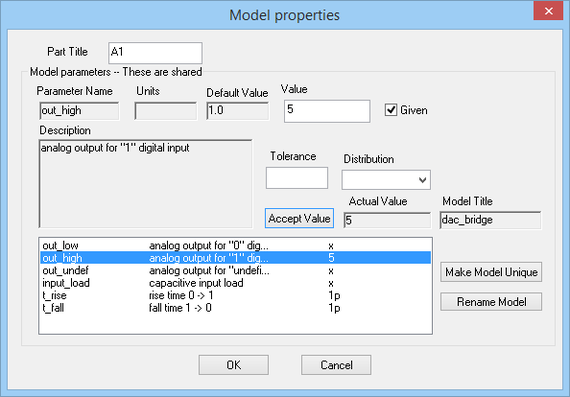Setting the output voltage levels and rise/fall times of the DAC conversion bridge.

You can use the circuit of the previous part and simply replace the old analog pulse source with the new digital source and the DAC bridge as shown below. Also, open the property dialog of the T-Line XTL1 and set its Alpha parameters to zero to have a lossless line.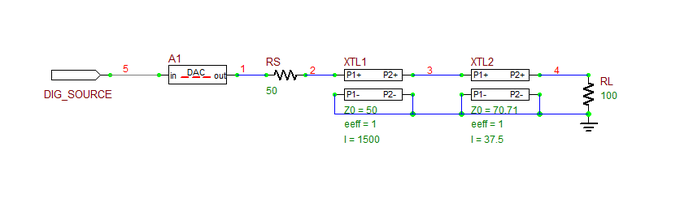A digital source connected to a long transmission line segment.

Run a Transient Test of this circuit with the parameters specified below:

 Start Time 0 20n 1p 1p v(2), v(4), dig_source

The results are shown in the figure below. The yellow binary input pulse train has a total duration of 8ns, and the input voltage v(2) drops to zero after t = 8ns. The transmitted pulse train reaches the load at t = 5.125ns. The reflected signal from the load reaches Node 2 at t = 10.25ns, when you see v(2) rise once again, and it continues until t = 18.25ns. Also note the sizable distortion of the transmitted signal at the load.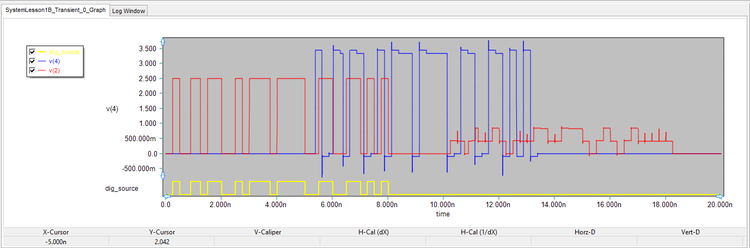The graphs of input voltage v(2), output voltage v(4) and the binary output of the digital source.

## Exploring Pulse Width Modulation

The following is a list of parts needed for this part of the tutorial lesson:

Part Name Part Type Part Value
VS Voltage Source Waveform: TBD
R1 Resistor 50
X1 Pulse Width Modulation Block Defaults
X2 Ramp Generator Defaults

A pulse width modulator (PWM) generates the binary data from an analog signal. It has a analog signal input (vs) and another input for a ramp generator (vramp). The PWM basically consists of a voltage comparator that compares the ramp and input signals. The binary output is given by:

$v_{out} = \begin{array}{ll} V_{high}, & v_s \gt v_{ramp} \\ V_{low}, & v_s \lt v_{ramp} \end{array}$

Place and connect the parts as shown in the figure below. You can access the Ramp Generator from the menu item Menu > Parts > Waveform Generation Blocks > Basic Waveforms > Ramp Generator. Open its property dialog and set its Period to 1ns. Set the out_high and out_low voltage levels to +2V and 0V, respectively. You can access the PWM modulator from the menu item Menu > Parts > Modulation Blocks > Pulse Width Modulation (PWM) Block. Open its property dialog and set its T_rmp (ramp period) to 1ns. Set the values of rmp_high and rmp_low parameters (input ramp min and max levels) to +2V and 0V, respectively. You have to make sure that these parameters are consistent. Keep all the other default parameter values.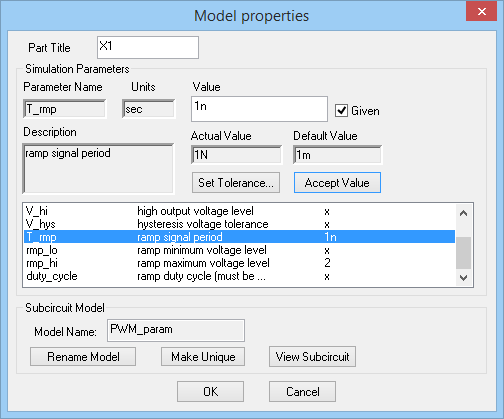The property dialog of a PWM modulator.The property dialog of a ramp generator.

Define a sinusoidal waveform for your voltage source according to the table below:

 Offset Voltage 1 1 100Meg 0 0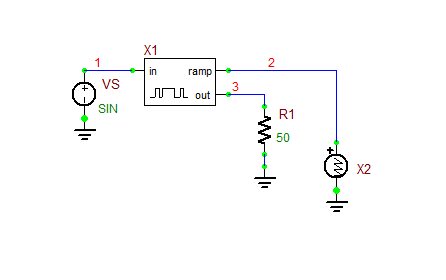A basic circuit to test a PWM modulator.

Run a Transient Test of this circuit with the parameters specified below:

 Start Time 0 30n 1p 1p v(1), v(2), v(3)

The results are shown in the figure below. Note that even though the output voltage has a binary nature, switching between the low and high levels, the output of the PWM block is an analog signal.

## Transmitting a PWM Signal Over a Long Distance

The following is a list of parts needed for this part of the tutorial lesson:

Part Name Part Type Part Value
VS Voltage Source Waveform: TBD
XTL1 Generic T-Line Defaults: Z0 = 50, eeff = 1, len = 1500
XTL2 Generic T-Line Defaults: Z0 = 70.71, eeff = 1, len = 37.5
RS Resistor 50
RL Resistor 100
X1 T_rmp = 1n, rmp_low = 0, rmp_high = 2 Defaults
X2 Ramp Generator period = 1n, out_low = 0, out_high = 2

In this part of the tutorial lesson, you will use the PWM modulator of the previous part to feed a binary input signal to the transmission line circuit of the first part. place and connect all the parts as shown in the figure below: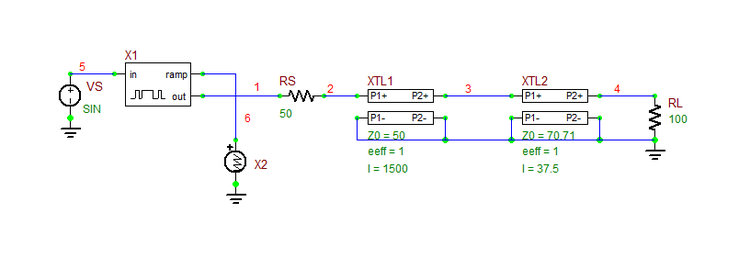Transmitting the binary output of a PWM modulator through a long transmission line segment.

Use the same sinusoidal waveform definition for the voltage source VS:

 Offset Voltage 1 1 100Meg 0 0

Run a Transient Test of this circuit with the parameters specified below:

 Start Time 0 30n 1p 1p v(1), v(2), v(4)

The results are shown in the figure below. All the three graphs are initially plotted together on a single chart. You can separate the graphs by activating the graph window first and selecting the menu item Menu > Edit> Separate Plots With Vertical Tiling. The voltage v(1) is the output of the PWM modulator and the same as you saw before in the previous part. You can see from the figure that the output voltage v(4) at the load is visibly distorted. But it still preserves the transmitted data reasonably well.Three separate plots for v(1), v(2) and v(4) with a periodic sinusoidal input signal.

## Defining Complex Input Waveforms

The following is a list of the additional parts needed for this part of the tutorial lesson:

Part Name Part Type Part Value
VP Voltage Source Waveform: TBD
B1 Nonlinear Controlled Voltage Source Definition: TBD

So far you have mostly used SPICE's standard voltage sources with a few waveforms like constant, sinusoidal and pulse. In the last part of this tutorial lesson, you will use RF.Spice's "Nonlinear Controlled Source" to synthesize more complex waveforms. For example, your input signal to the PWM modulator in the previous part was a periodic sinusoid. In this part, you will use a time-limited sinusoid with a finite duration of 8ns. You can make this waveform by multiplying the original sinusoid by a one-shot pulse of the same duration. The latter can be defined as a pulse waveform with an extremely large period. Place the new voltage source VP and define its waveform as specified in the table below:

 Offset Voltage 1 1 100Meg 0 0

 Initial Voltage 0 1 0 0 0 8n 1e3

You can place the nonlinear source using the keyboard shortcut B. Define its voltage as the product of the voltages of VS and VP as shown in the figure below: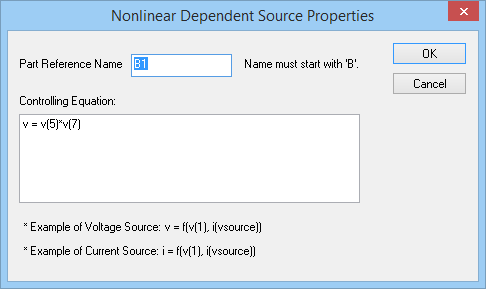The property dialog of the Nonlinear Controlled Voltage Source.

Your new circuit should like this:The PWM modulator with a long transmission line and a nonlinear controlled signal source.

Run a Transient Test of this circuit with the parameters specified below:

 Start Time 0 20n 1p 1p v(1), v(2), v(4), v(8)

The results are shown in the figure below. Note that there is no source input to the transmission line after t = 8ns. The size of the reflected signal returning to Node 2 is significant.Three separate plots for v(1), v(2) and v(4) with a time-limited sinusoidal input signal.

Finally, change the waveform of VP to exponential and change the sinusoidal waveform of VS according to the table below:

 Offset Voltage 1 1 200Meg 0 0

 Initial Voltage 0 1 0 1n 12n 0.1n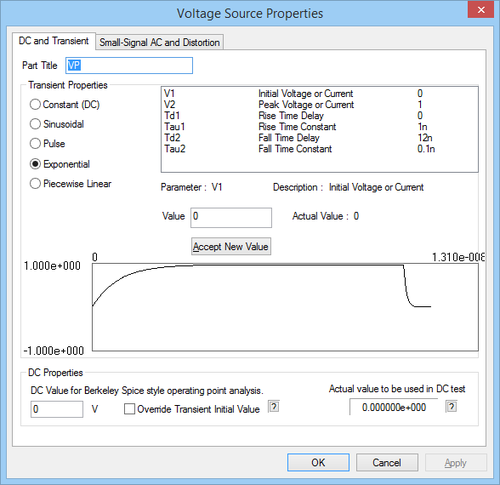The property dialog of the voltage source VP with an exponential waveform.

Run a Transient Test of this circuit with the parameters specified below:

 Start Time 0 25n 1p 1p v(1), v(2), v(4), v(8)

The results are shown in the figure below. Note that the output of the PWM modulator has completely changed from the previous cases due to an entirely different waveform. While highly distorted, the output signal at the load still maintains the digital data. The input signal at Node 2 is identical to the input PWM signal until t = 10.25ns, when the reflected signal from the load strikes. The figure also shows the input analog signal at Node 8. After t = 12.18ns, both v(8) and v(1) drop to zero.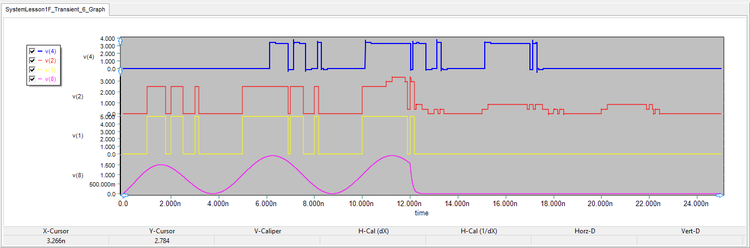Four separate plots for v(1), v(2), v(4) and v(8) with a complex input signal.